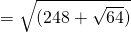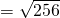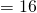Square root

Square root

If you have to find square of a number then we write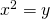it means we multiplied x two times and got y.

For example find the square of 2 that you have to multiple 2 two timesWe find square of a number by multiplying that number twice.

So what is a square root

Finding a square root is reverse process of square.

In square root we find the number which is multiplied itself to get the square of number.

a square root is represented by symbolThis symbol is known as square root.

here x represents the number whose square root we want to find

In general

ifthen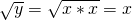,in above example

Square root of y is x
square root of 4 is 2
square root of 9 is 3

Example find the value of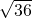We all know 36 is square of 6

so we can write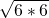so=Find the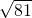\sqrt{9*9}=9

Finde the square root of 625

by factoring 625

we get 625=5*5*5*5

so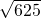=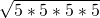=5*5

=25

To find the square root of 2 is as below

Cube root

We can find cube of a number by multiplying it three times

for example to find cube root of x we multiply it tree times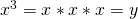Here y is cube of x

Finding the cube of 2

that is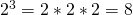here 8 is cube of 2

similar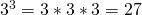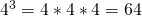Now what is cube root

Cube root is a reverse process of a cube.

In cube root we find the number which is multiplied itself thrice to get the cube of number.here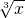is known as cube root ofCube root is represented by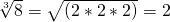here x is number whose cube root we want to fine

Finding Square & cube root by factorization

Find th square root of 6084Calculate the value of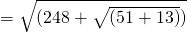169 is square of 13